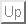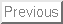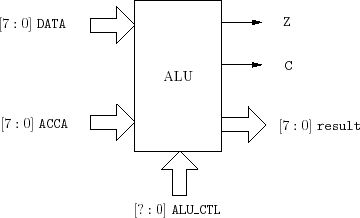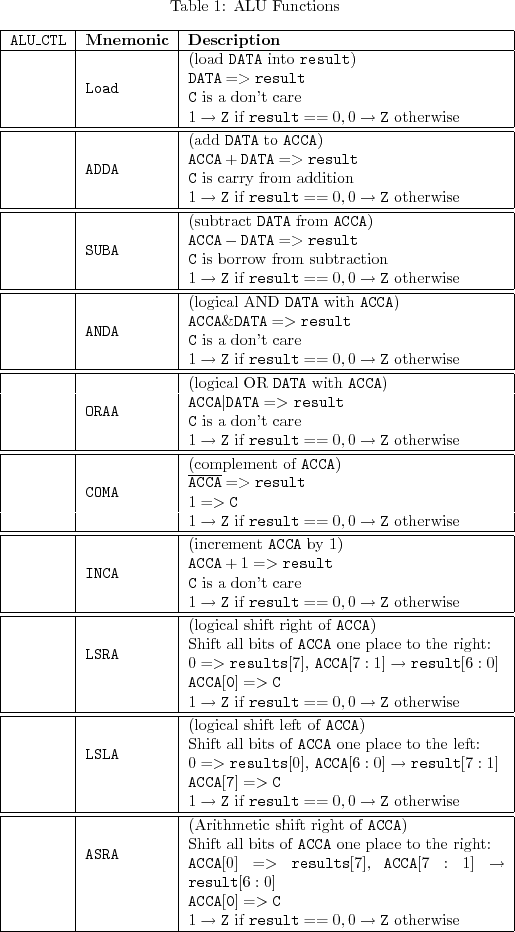Next: 1 Prelab

# Lab 5: Arithmetic Logic Unit (ALU)

The heart of every computer is an Arithmetic Logic Unit (ALU). This is the part of the computer which performs arithmetic operations on numbers, e.g. addition, subtraction, etc. In this lab you will use the Verilog language to implement an ALU having 10 functions. Use of the case structure will make this job easy.The ALU that you will build (see Figure 1) will perform 10 functions on 8-bit inputs (see Table 1). Please make sure you use the same variable name as the ones used in this lab. Don't make your own. The ALU will generate an 8-bit result (result), a one bit carry (C), and a one bit zero-bit (Z). To select which of the 10 functions to implement you will use ALU_CTL as the selection lines.